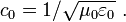# Free space (electromagnetism)

Free space usually refers to a perfect vacuum, devoid of all particles. The term is most often used in classical electromagnetism where it refers to a reference state sometimes called classical vacuum, and in quantum physics where it refers to the ground state of the electromagnetic field, which is subject to fluctuations about a dormant zero average-field condition. The classical case of vanishing fields implies all fields are source-attributed, while in the quantum case field moments can arise without sources by virtual photon creation and destruction. The description of free space varies somewhat among authors, with some authors requiring only the absence of substances with electrical properties, or of charged matter (ions and electrons, for example).

## Contents

### Classical case

In classical physics, free space is a concept of electromagnetic theory, corresponding to a theoretically perfect vacuum and sometimes referred to as the vacuum of free space, or as classical vacuum, and is appropriately viewed as a reference medium. In the classical case, free space is characterized by the electrical permittivity ε0 and the magnetic permeability μ0. The exact value of ε0 is provided by NIST as the electric constant  and the defined value of μ0 as the magnetic constant:

ε0 ≈ 8.854 187 817... × 10−12 F m−1
μ0 = 4π × 10−7 ≈ 12.566 370 614... x 10−7 N A−2

where the approximation is not a physical uncertainty (such as a measurement error) but a result of the inability to express these irrational numbers with a finite number of digits.

One consequence of these electromagnetic properties coupled with Maxwell's equations is that the speed of light in free space is related to ε0 and μ0 via the relation:$c_0 = 1/\sqrt{\mu_0 \varepsilon_0}\ .$

Using the defined valued for the speed of light provided by NIST as:

c0 = 299 792 458 m s −1,

and the already mentioned defined value for μ0, this relationship leads to the exact value given above for ε0.

Another consequence of these electromagnetic properties is that the ratio of electric to magnetic field strengths in an electromagnetic wave propagating in free space is an exact value provided by NIST as the characteristic impedance of vacuum:$Z_0 = \sqrt{\mu_0 /\varepsilon_0} \$
= 376.730 313 461... Ω.

It also can be noted that the electrical permittivity ε0 and the magnetic permeability μ0 do not depend upon direction, field strength, polarization, or frequency. Consequently, free space is isotropic, linear, non-dichroic, and dispersion free. Linearity, in particular, implies that the fields and/or potentials due to an assembly of charges is simply the addition of the fields/potentials due to each charge separately (that is, the principle of superposition applies).

### Quantum case

For a discussion of the quantum case, see Vacuum (quantum electrodynamic).

### Attainability

A perfect vacuum is itself only realizable in principle. It is an idealization, like absolute zero for temperature, that can be approached, but never actually realized:

 “One reason [a vacuum is not empty] is that the walls of a vacuum chamber emit light in the form of black-body radiation...If this soup of photons is in thermodynamic equilibrium with the walls, it can be said to have a particular temperature, as well as a pressure. Another reason that perfect vacuum is impossible is the Heisenberg uncertainty principle which states that no particles can ever have an exact position...More fundamentally, quantum mechanics predicts ... a correction to the energy called the zero-point energy [that] consists of energies of virtual particles that have a brief existence. This is called vacuum fluctuation.” Luciano Boi, "Creating the physical world ex nihilo?" p. 55

And classical vacuum is one step further removed from attainability because its permittivity ε0 and permeability μ0 do not allow for quantum fluctuations. Nonetheless, outer space and good terrestrial vacuums are modeled adequately by classical vacuum for many purposes.